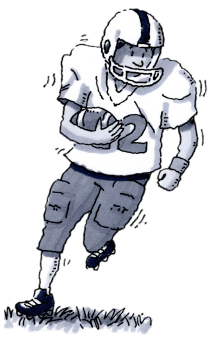### Home > CC2MN > Chapter 6 Unit 6B > Lesson CC2: 6.2.2 > Problem6-70

6-70.To solve the following problem, use the 5-D Process. Define a variable and write an expression for each column of your table.

In the first three football games of the season, Carlos gained three times as many yards as Alston. Travis gained ten yards more than Carlos. Altogether, the three players gained a total of $430$ yards. How many yards did Carlos gain? Homework Help ✎

Based on the information from the problem, two of the players, Carlos and Travis, can be directly related to Alston in yardage. If Alston's yards are represented by the variable, $x$, how can Carlos and Travis' yards be represented?

Now run some trials of your own to determine how many yards Carlos gained.

 Define Do Decide
 Define Do Decide Alston’s yards$x$ Carlos’s yards$3x$ Travis’s yards$3x+10$ Sum of the yards$x+3x+\left(3x+10\right)$ $430$ yards
 Define Do Decide Alston’s yards$x$ Carlos’s yards$3x$ Travis’s yards$3x+10$ Sum of the yards$x+3x+\left(3x+10\right)$ $430$ yards $50$ $150$ $160$ $50+50+160$ $360$ yardsToo Low
 Define Do Decide Alston’s yards$x$ Carlos’s yards$3x$ Travis’s yards$3x+10$ Sum of the yards$x+3x+\left(3x+10\right)$ $430$ yards $50$ $70$ $150$  $210$ $160$ $220$ $50+50+160$ $70+210+220$ $360$ yardsToo Low$500$ yardsToo High